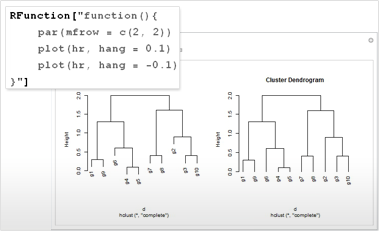# Built-in Integration with R

Mathematica 9 offers built-in ways to integrate R code into your Mathematica workflow, combining Mathematica's broad range of capabilities with the statistical computing language. RLink uses J/Link and rJava/JRI Java libraries to allow the user to exchange data between Mathematica and R and to execute R code from within Mathematica. With RLink, R users can use thousands of functions from across the full Mathematica system.• Full access to all R functionality from within Mathematica. »
• Automatically download an R runtime on any platform or use your own R distribution on Windows.
• Exchange data between Mathematica and R, using the usual Mathematica representation for the data—most core R data types are supported. »
• Create functions in the R workspace from within Mathematica. »
• Apply built-in and user-defined R functions from within Mathematica in a way natural for Mathematica users.
• Seamless application of built-in and user-defined R functions on Mathematica data, supporting all kinds of R functions, including closures and higher-order functions.
• Extend the core RLink data types as needed with user-defined types for specialized use.
• Build interactive applications for analyzing and viewing data with Mathematica's instant interactive exploration and automatic interface construction. »
• Analyze in R and display results with high-quality static or dynamic representations powered by Mathematica's visualization engine. »

## Mathematica

Questions? Comments? Contact a Wolfram expert »